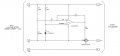# Comparator Hystersis for Low Voltage Disconnect

#### wd8cxb

Joined Jul 8, 2009
10
I designed a Low Voltage Disconnect for a 3 Cell Li-Ion Battery pack. Nominal voltage being 10.8V. I set the LVD to turn off the output at 9V. The charger used for this battery pack will not recharge a battery if the voltage is below 7.5V. If the battery is left on the shelf for a period of time I want to have a couple volts available to power the LVD circuit.

The battery back powers a device with a motor and embedded microcontroller. I need to implement some type of hysteresis and if controlling the output with a P Channel MOSFET it would be rather easy as I can just put a resister between the common rail and the voltage reference and then add a feedback resistor to the cathode of voltage reference and the output of the op-amp. When the output is off it would add a few millivolts to the reference point and then lower the reference voltage when the LVD is on.

The circuit I have shown below might work for my application but I am having trouble picking the correct values for R5 or R6. I am not sure if this is the best way of having a circuit that turns on at 10.8V and above while turning off when the voltage is at 9VAny recommendations for circuit modifications or possibly a formula to calculate the proper resistor values to make this work as intended?

#### Dodgydave

Joined Jun 22, 2012
10,082
Your resistor R6, sets the Hysteresis, as you have the reference point set at 2.5V, the resistor R3 needs to be 3M, this will give you a trip voltage of 2.5V on pin 3, if you make R6 100M, the hysteresis will be +/- 0.1V.

Ideally i would use a preset resistor to fine tune the trip point for R6, and set it permanent when testing.

Last edited: